# NCERT Solutions for Class 7 Maths Chapter 15 – Visualising Solid Shapes

Class 7 Maths NCERT Solutions Chapter 15 – Visualising Solid Shapes comprises of the 4 Exercises

This Chapter contains the Exercises relating to the following topics , which are discussed in Chapter 14 – Symmetry Class 7 NCERT book  : –

• 15.1 INTRODUCTION: PLANE FIGURES AND SOLID SHAPES
• 15.2 FACES, EDGES AND VERTICES
• 15.3 NETS FOR BUILDING 3-D SHAPES
• 15.4 DRAWING SOLIDS ON A FLAT SURFACE
• 15.4.1 Oblique Sketches
• 15.4.2 Isometric Sketches
• 15.4.3 Visualising Solid Objects
• 15.5 VIEWING DIFFERENT SECTIONS OF A SOLID
• 15.5.1 One Way to View an Object is by Cutting or Slicing
• 15.5.2 Another Way is by Shadow Play
• 15.5.3 A Third Way is by Looking at it from Certain Angles to Get Different Views

### NCERT Solutions for Class 7 Maths Chapter 15 Exercise 15.1 – Visualising Solid Shapes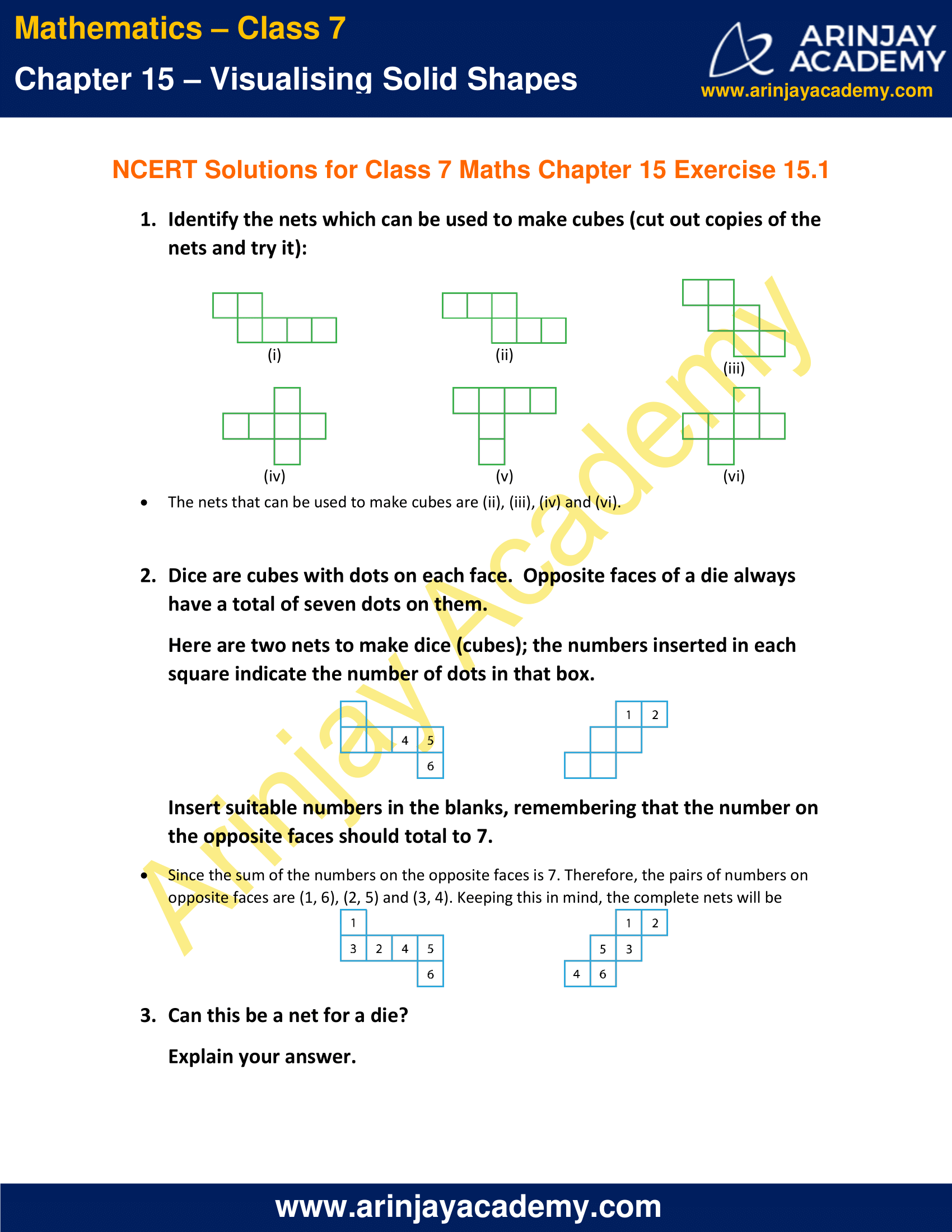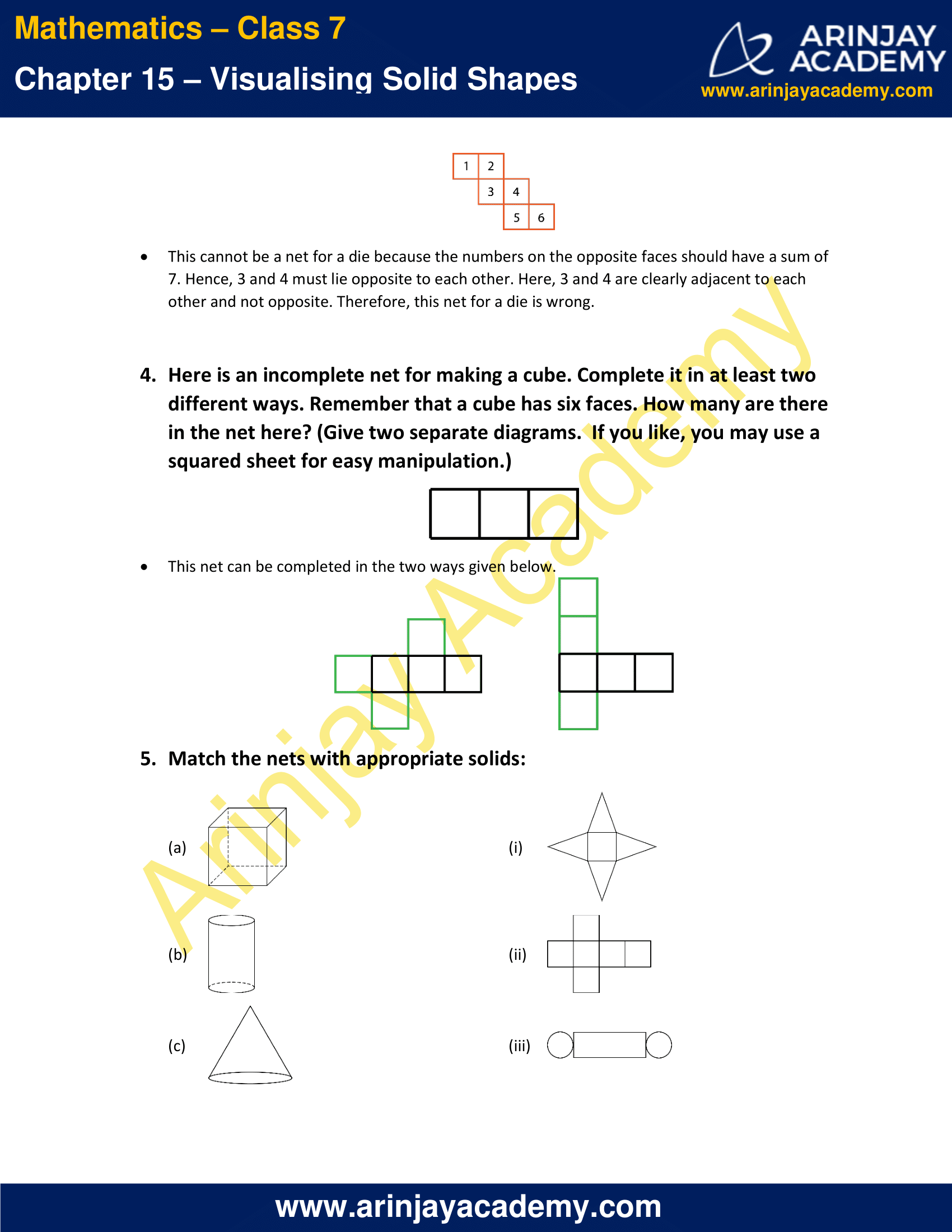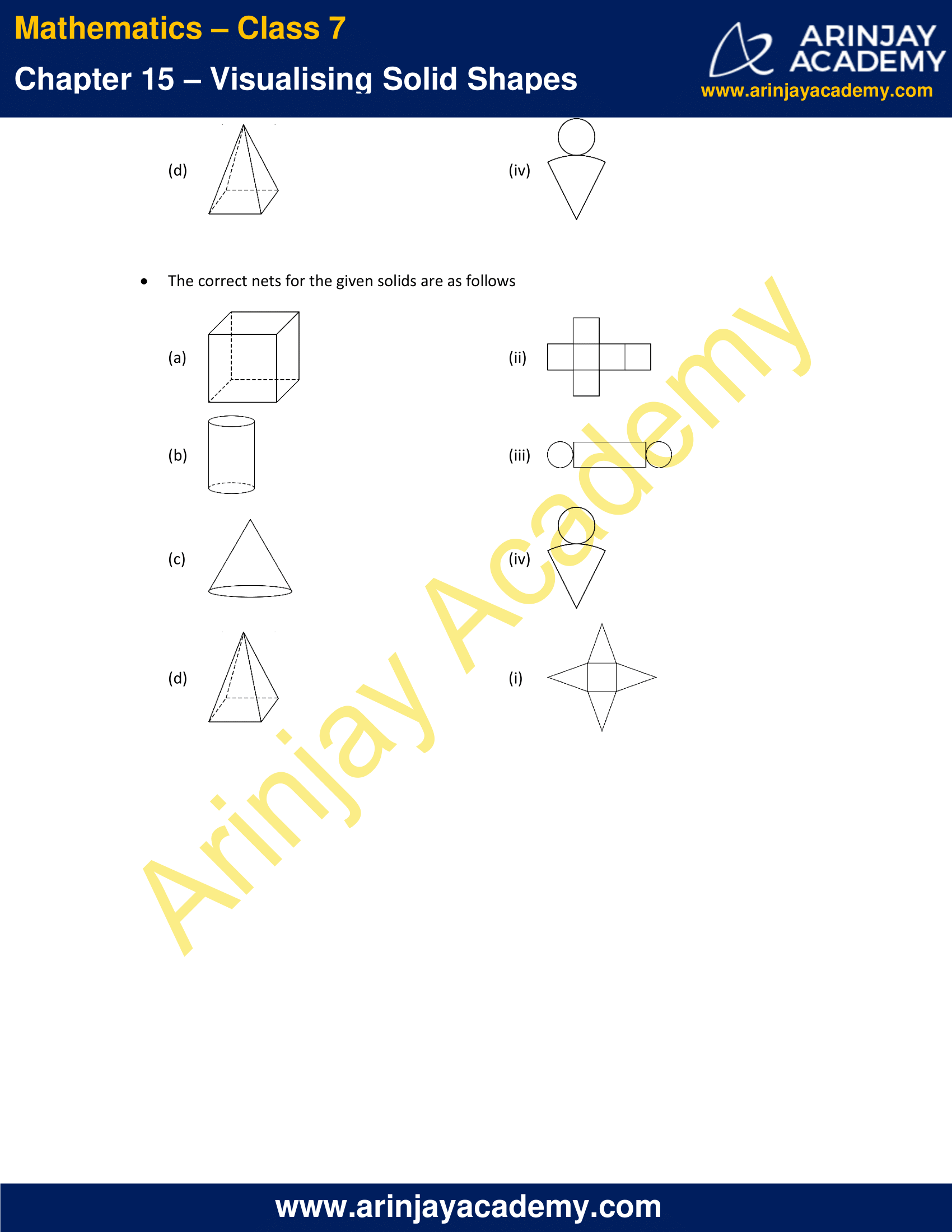### NCERT Solutions for Class 7 Maths Chapter 15 Exercise 15.2 – Visualising Solid Shapes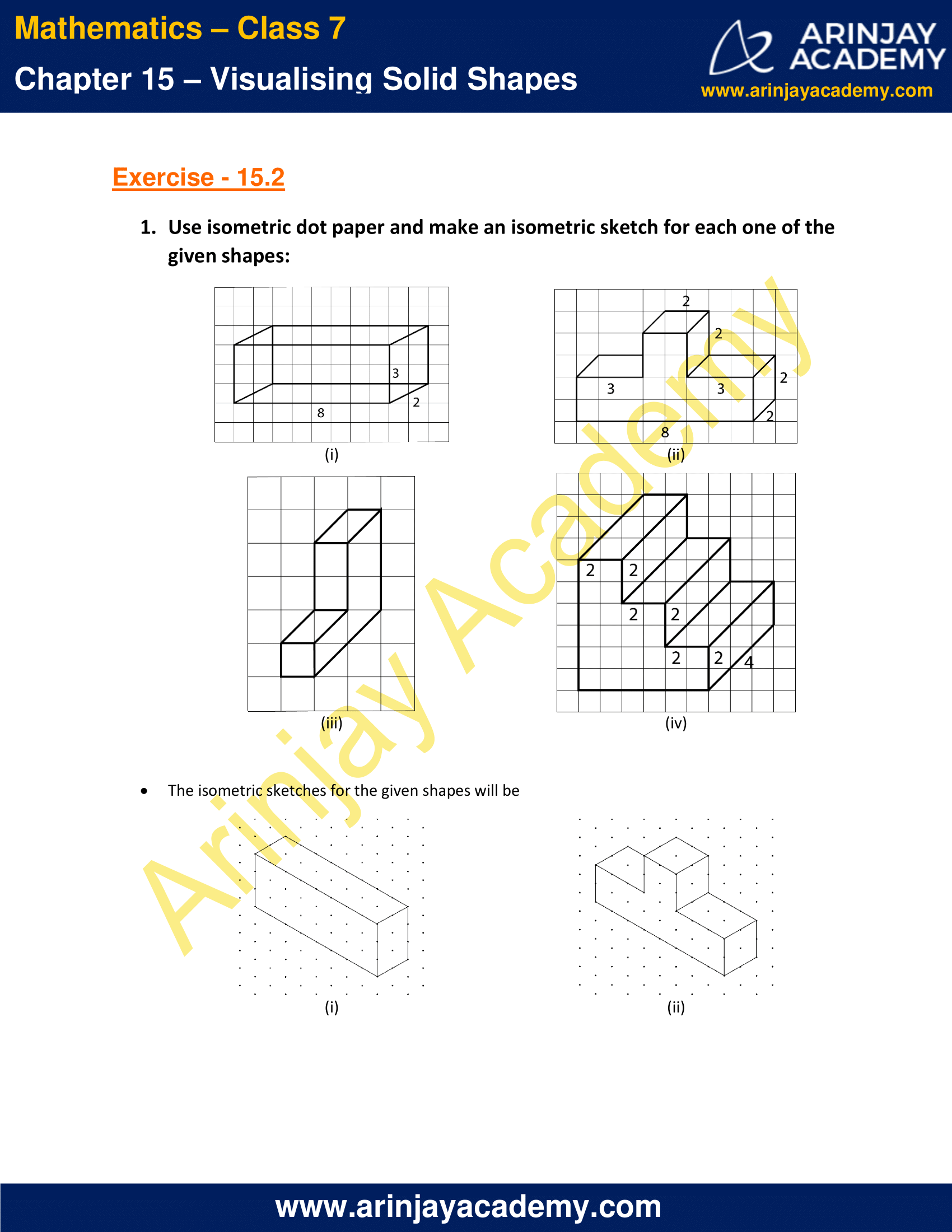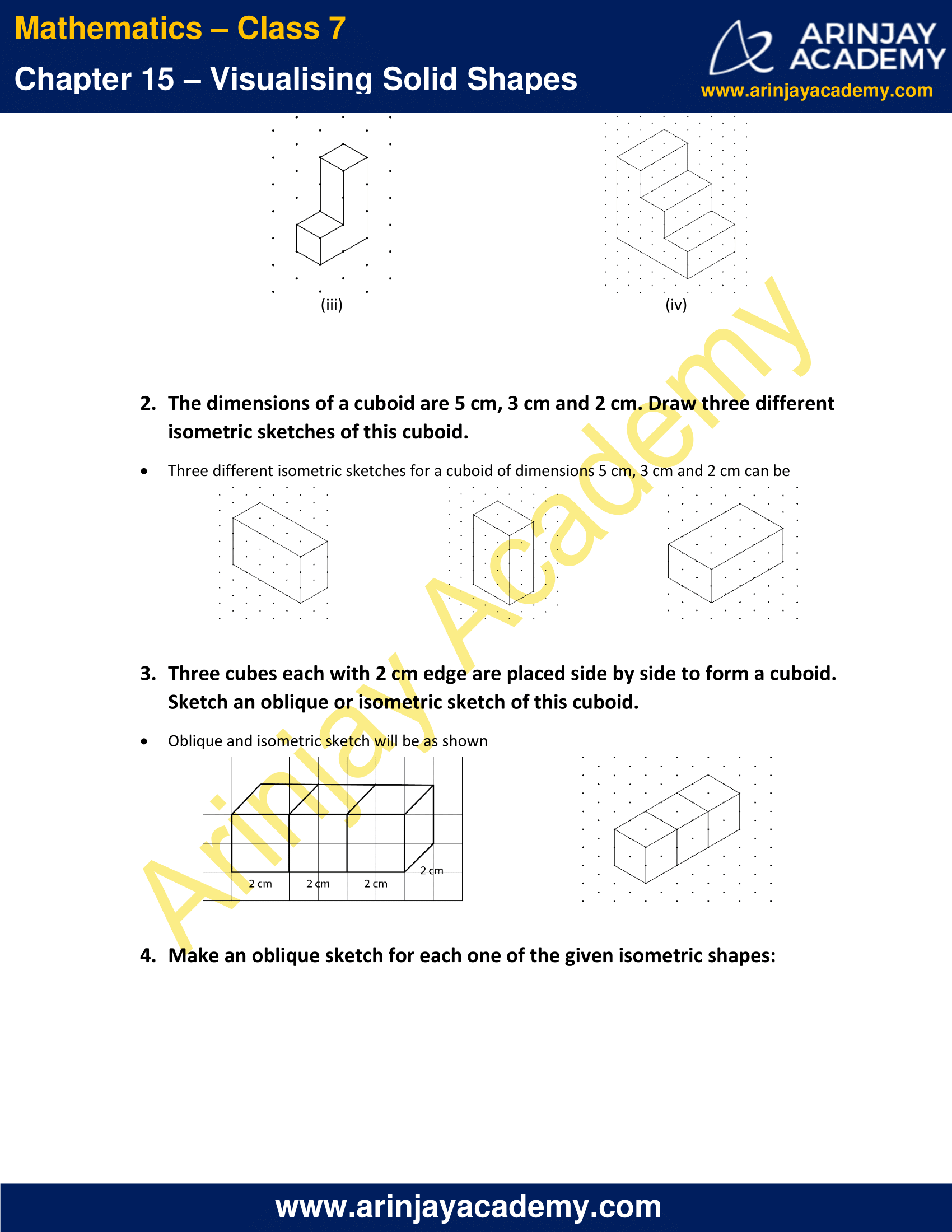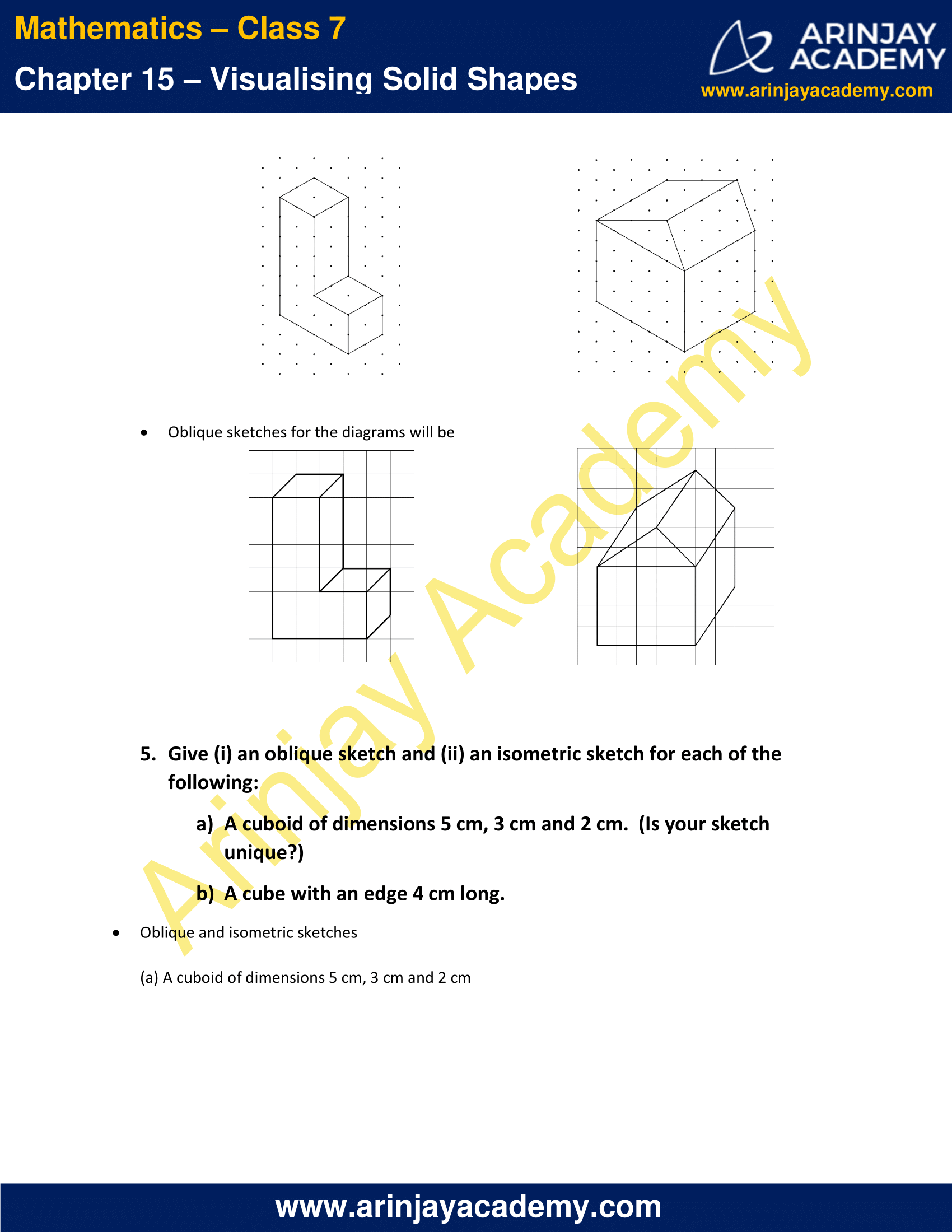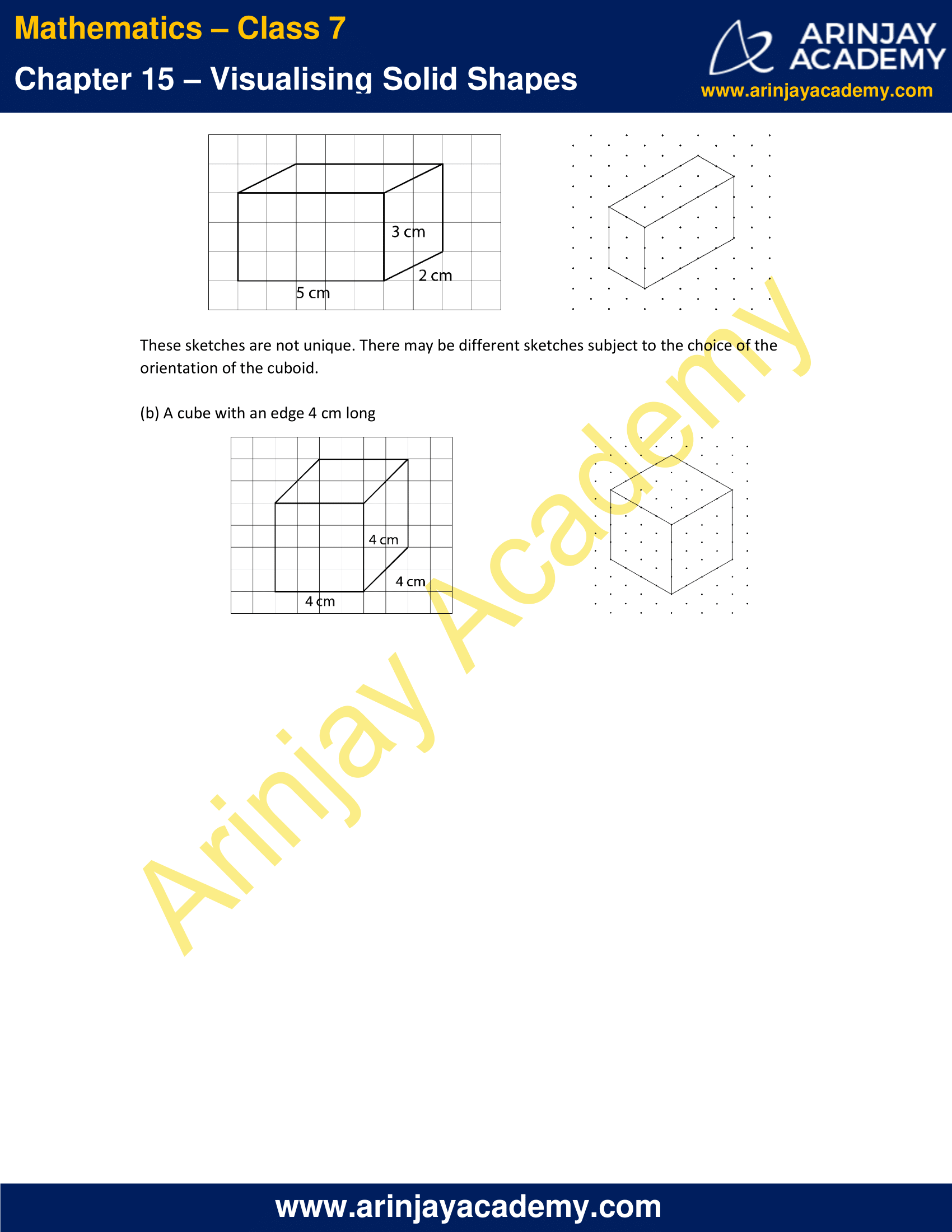### NCERT Solutions for Class 7 Maths Chapter 15 Exercise 15.3 – Visualising Solid Shapes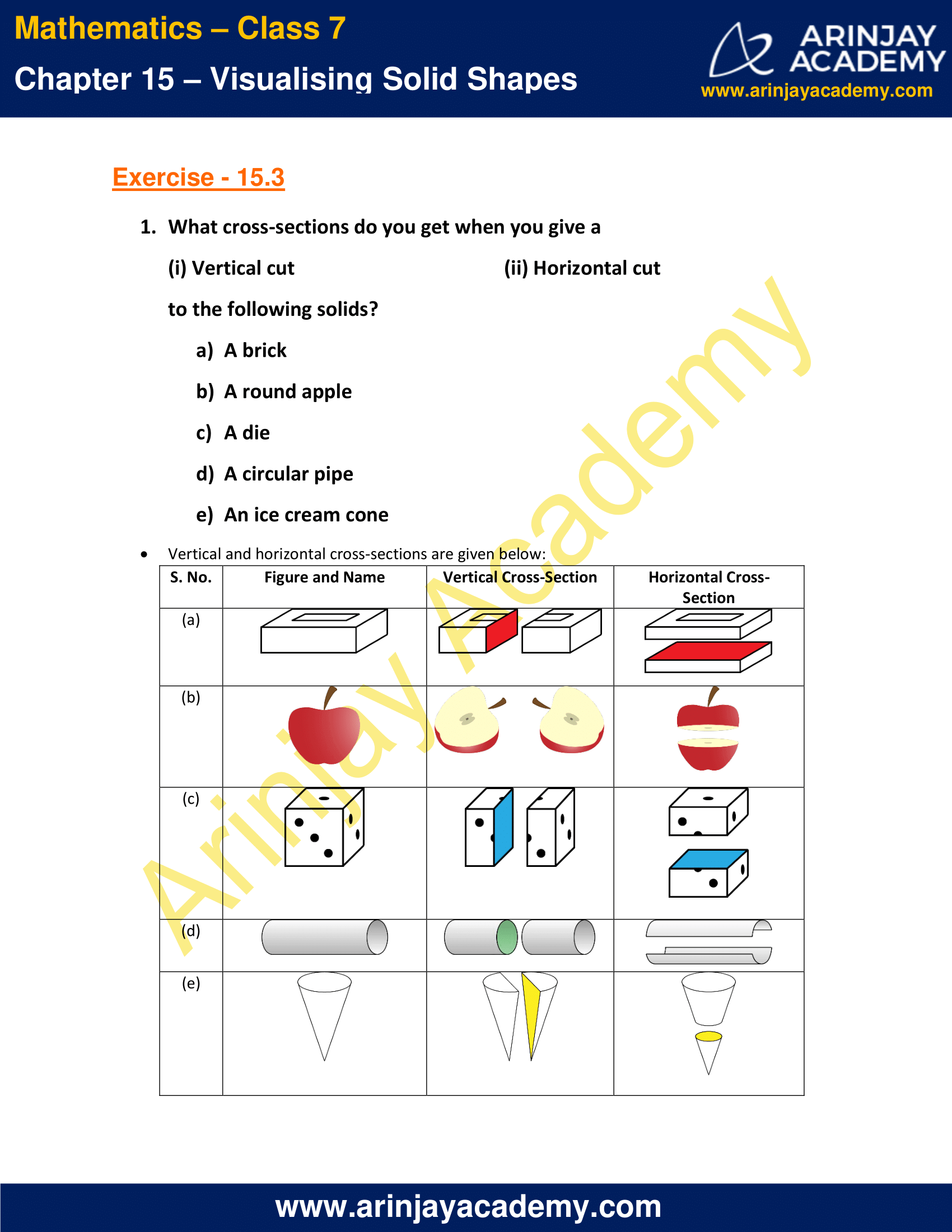### NCERT Solutions for Class 7 Maths Chapter 15 Exercise 15.4 – Visualising Solid Shapes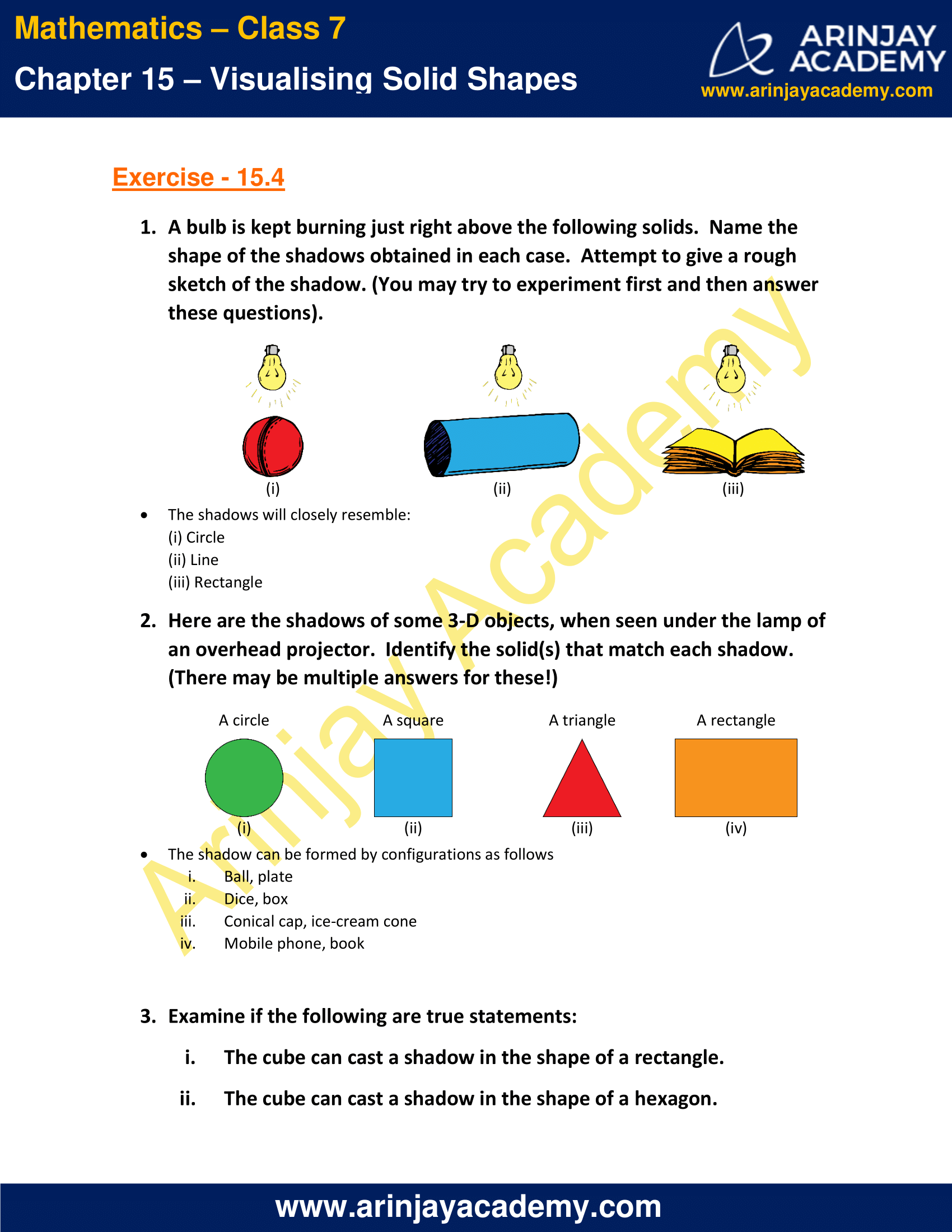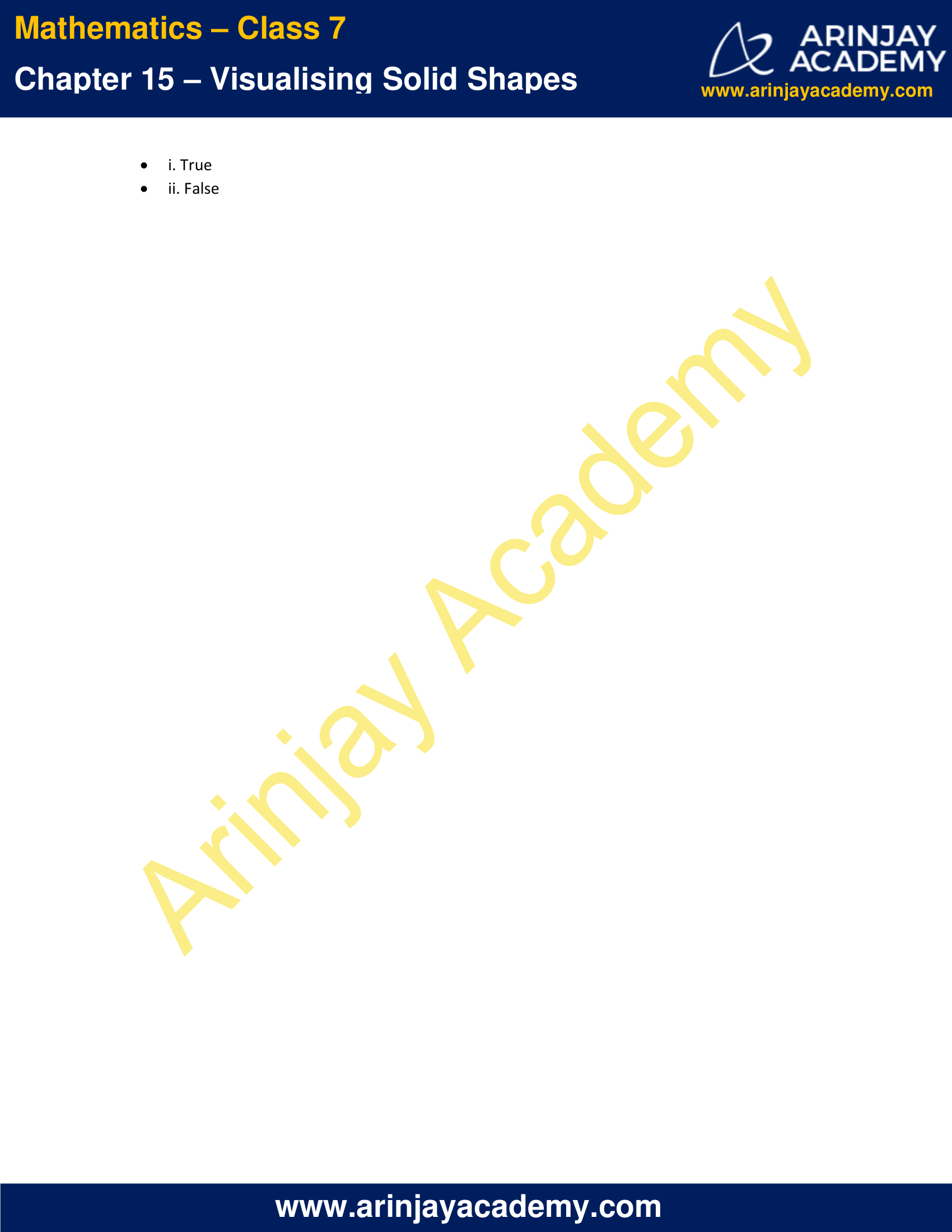### NCERT Solutions for Class 7 Maths Chapter 15 Exercise 15.1 – Visualising Solid Shapes

1. Identify the nets which can be used to make cubes (cut out copies of the nets and try it):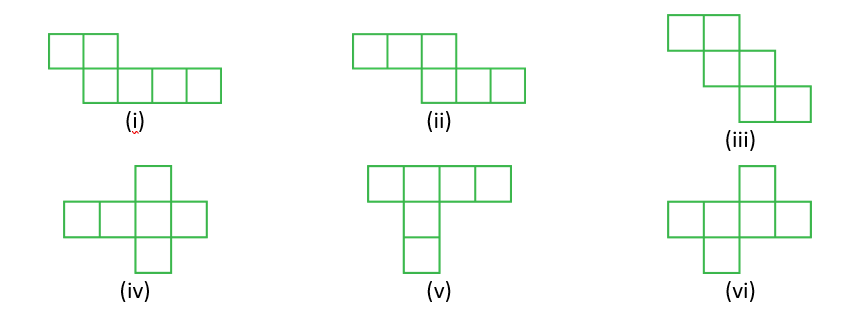Solution:

The nets that can be used to make cubes are (ii), (iii), (iv) and (vi).

2. Dice are cubes with dots on each face. Opposite faces of a die always have a total of seven dots on them.

Here are two nets to make dice (cubes); the numbers inserted in each square indicate the number of dots in that box.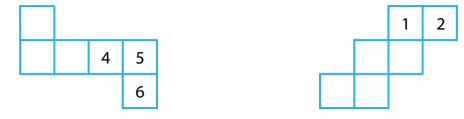Insert suitable numbers in the blanks, remembering that the number on the opposite faces should total to 7.

Solution:

Since the sum of the numbers on the opposite faces is 7. Therefore, the pairs of numbers on opposite faces are (1, 6), (2, 5) and (3, 4). Keeping this in mind, the complete nets will be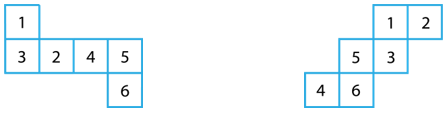3. Can this be a net for a die?
Explain your answer.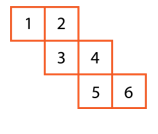Solution:

This cannot be a net for a die because the numbers on the opposite faces should have a sum of 7. Hence, 3 and 4 must lie opposite to each other. Here, 3 and 4 are clearly adjacent to each other and not opposite. Therefore, this net for a die is wrong.

4. Here is an incomplete net for making a cube. Complete it in at least two different ways. Remember that a cube has six faces. How many are there in the net here? (Give two separate diagrams. If you like, you may use a squared sheet for easy manipulation.)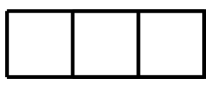Solution:

This net can be completed in the two ways given below.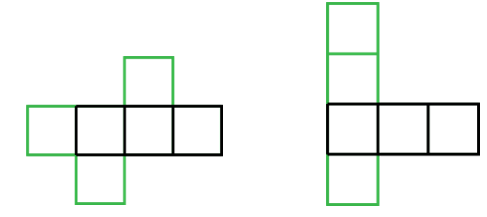5. Match the nets with appropriate solids: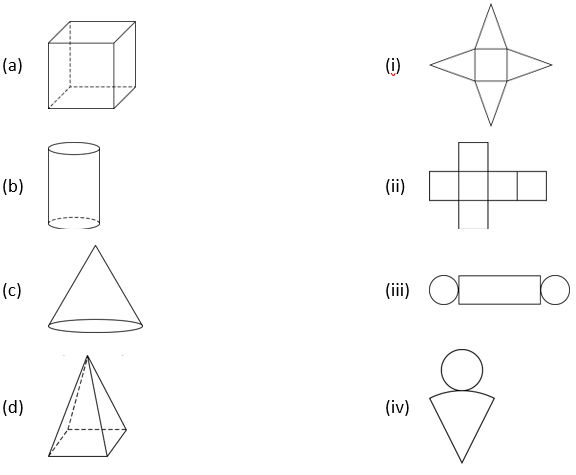Solution:

The correct nets for the given solids are as follows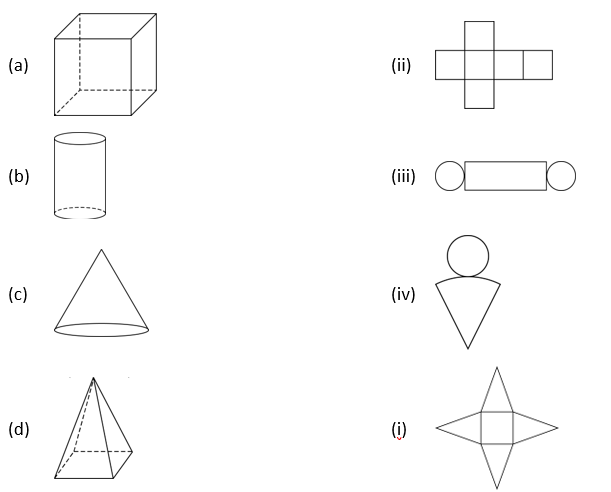### NCERT Solutions for Class 7 Maths Chapter 15 Exercise 15.2 – Visualising Solid Shapes

1. Use isometric dot paper and make an isometric sketch for each one of the given shapes: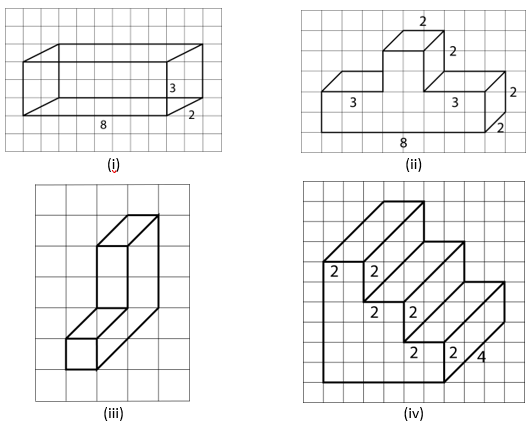Solution:

The isometric sketches for the given shapes will be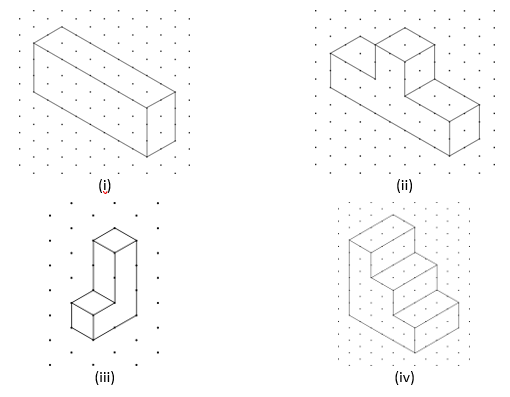2. The dimensions of a cuboid are 5 cm, 3 cm and 2 cm. Draw three different isometric sketches of this cuboid.

Solution:

Three different isometric sketches for a cuboid of dimensions 5 cm, 3 cm and 2 cm can be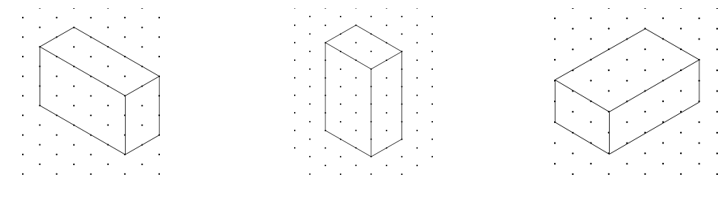3. Three cubes each with 2 cm edge are placed side by side to form a cuboid. Sketch an oblique or isometric sketch of this cuboid.

Solution:

Oblique and isometric sketch will be as shown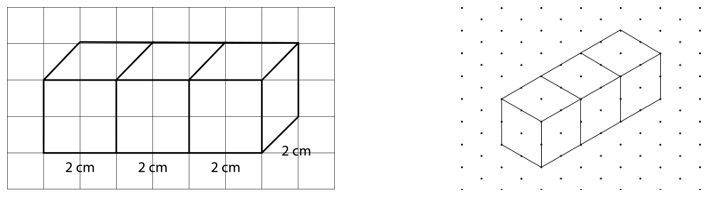4. Make an oblique sketch for each one of the given isometric shapes: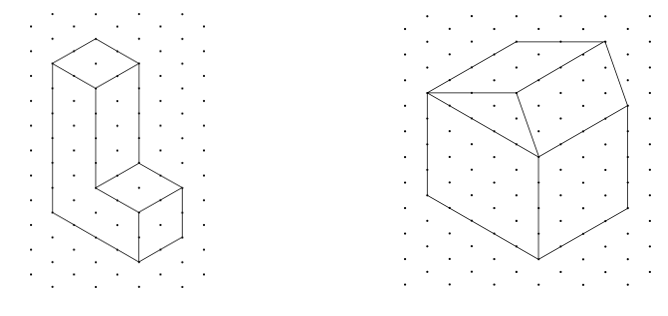Solution:

Oblique sketches for the diagrams will be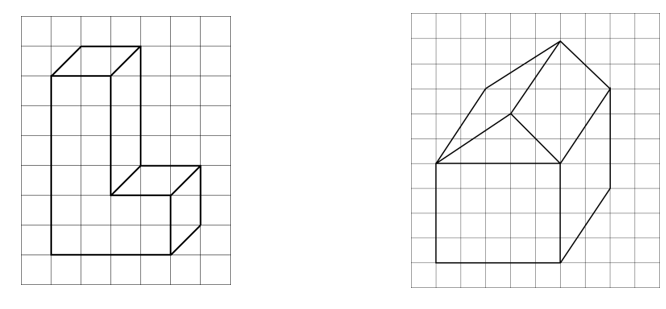5. Give (i) an oblique sketch and (ii) an isometric sketch for each of the following:

a) A cuboid of dimensions 5 cm, 3 cm and 2 cm. (Is your sketch unique?)
b) A cube with an edge 4 cm long.

Solution:

Oblique and isometric sketches

(a) A cuboid of dimensions 5 cm, 3 cm and 2 cm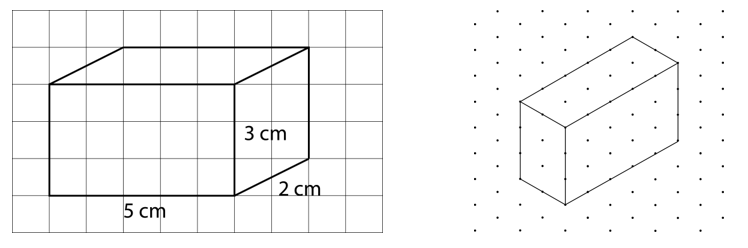These sketches are not unique. There may be different sketches subject to the choice of the orientation of the cuboid.

(b) A cube with an edge 4 cm long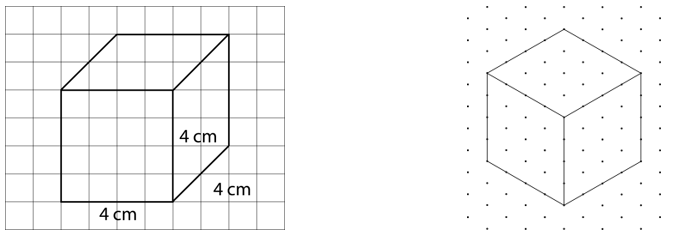### NCERT Solutions for Class 7 Maths Chapter 15 Exercise 15.3 – Visualising Solid Shapes

1. What cross-sections do you get when you give a
(i) Vertical cut
(ii) Horizontal cut
to the following solids?

a) A brick
b) A round apple
c) A die
d) A circular pipe
e) An ice cream cone

Solution:

Vertical and horizontal cross-sections are given below:

### NCERT Solutions for Class 7 Maths Chapter 15 Exercise 15.4 – Visualising Solid Shapes

1. A bulb is kept burning just right above the following solids. Name the shape of the shadows obtained in each case.  Attempt to give a rough sketch of the shadow. (You may try to experiment first and then answer these questions).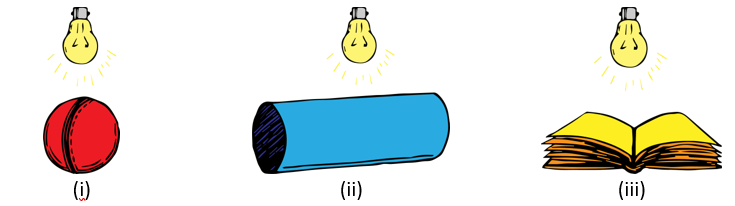Solution:

The shadows will closely resemble:
(i) Circle
(ii) Line
(iii) Rectangle

2. Here are the shadows of some 3-D objects, when seen under the lamp of an overhead projector. Identify the solid(s) that match each shadow. (There may be multiple answers for these!)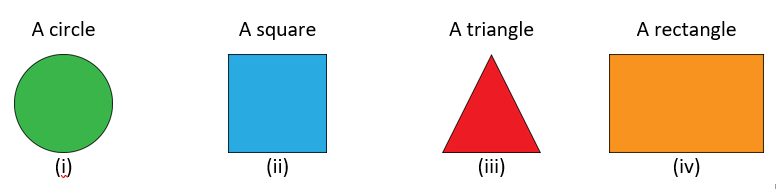Solution:

The shadow can be formed by configurations as follows

i. Ball, plate
ii. Dice, box
iii. Conical cap, ice-cream cone
iv. Mobile phone, book

3. Examine if the following are true statements:
i. The cube can cast a shadow in the shape of a rectangle.
ii. The cube can cast a shadow in the shape of a hexagon.

Solution:

i. True
ii. False

With this we come to the end of NCERT Solutions for Class 7 Maths Chapter 15 Visualising Solid Shapes. We hope these helped you study your subject.

Download NCERT Solutions for Class 7 Maths Chapter 15 – Visualising Solid Shapes